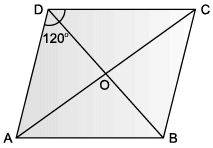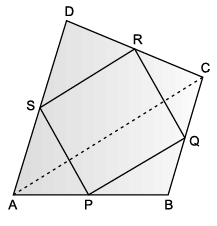Test: Quadrilaterals- Case Based Type Questions- 2

# Test: Quadrilaterals- Case Based Type Questions- 2 - Class 9

Test Description

## 10 Questions MCQ Test Additional Practice for Class 9 - Test: Quadrilaterals- Case Based Type Questions- 2

Test: Quadrilaterals- Case Based Type Questions- 2 for Class 9 2023 is part of Additional Practice for Class 9 preparation. The Test: Quadrilaterals- Case Based Type Questions- 2 questions and answers have been prepared according to the Class 9 exam syllabus.The Test: Quadrilaterals- Case Based Type Questions- 2 MCQs are made for Class 9 2023 Exam. Find important definitions, questions, notes, meanings, examples, exercises, MCQs and online tests for Test: Quadrilaterals- Case Based Type Questions- 2 below.
 1 Crore+ students have signed up on EduRev. Have you?
Test: Quadrilaterals- Case Based Type Questions- 2 - Question 1

### ABCD is an area in the shape of rhombus in which ∠ADC = 120°. Samay and Tarun lived at D and C and their school located at O.Q. Who can reach school early?

Detailed Solution for Test: Quadrilaterals- Case Based Type Questions- 2 - Question 1
∠CDO = 60° and ∠DCO = 30°

Since, ∠CDO > ∠ DCO

⇒ CO > DO.

(side opposite to greater angle is greater.)

⇒ Samay will reach school early.

Test: Quadrilaterals- Case Based Type Questions- 2 - Question 2

### ABCD is an area in the shape of rhombus in which ∠ADC = 120°. Samay and Tarun lived at D and C and their school located at O.Q. Measure of ∠CDO and ∠DCO are ______ and ______ respectively.

Detailed Solution for Test: Quadrilaterals- Case Based Type Questions- 2 - Question 2

Diagonal of a rhombus bisects the angles is passing from.

So,

∠CDO = 1/2 ∠CDA

= 1/2 (120)° = 60°

120° + ∠DCB = 180°

⇒ ∠DCB = 180° – 120° = 60°

∠DCO = 1/2 ∠DCB

= 1/2 (60°) = 30°

Test: Quadrilaterals- Case Based Type Questions- 2 - Question 3

### ABCD is an area in the shape of rhombus in which ∠ADC = 120°. Samay and Tarun lived at D and C and their school located at O.Q. Measure of ∠DCB is :

Detailed Solution for Test: Quadrilaterals- Case Based Type Questions- 2 - Question 3
ABCD is a rhombus and adjacent angles of a rhombus are supplementary.

Thus, ∠CDA + ∠DCB = 180°

⇒ 120° + ∠DCB = 180°

⇒ ∠DCB = 180° – 120° = 60°

Test: Quadrilaterals- Case Based Type Questions- 2 - Question 4

ABCD is an area in the shape of rhombus in which ∠ADC = 120°. Samay and Tarun lived at D and C and their school located at O.Q. Which statement is incorrect?

Detailed Solution for Test: Quadrilaterals- Case Based Type Questions- 2 - Question 4
Diagonals of a rhombus are not perpendicular bisectors.

Diagonals of a rhombus are perpendicular bisectors.

Test: Quadrilaterals- Case Based Type Questions- 2 - Question 5

ABCD is an area in the shape of rhombus in which ∠ADC = 120°. Samay and Tarun lived at D and C and their school located at O.Q. Measure of ∠BAC :

Detailed Solution for Test: Quadrilaterals- Case Based Type Questions- 2 - Question 5
Since, ABCD is a rhombus. So, AB||CD and AC is a transversal.

Thus, ∠BAC = ∠ DCA = 30° (alternate interior angles)

Test: Quadrilaterals- Case Based Type Questions- 2 - Question 6

Maths teacher of class 9th gave students coloured paper in the shape of quadrilateral and then ask the students to make parallelogram from it by using paper folding.Q. If S P = 3 cm, then RQ =

Detailed Solution for Test: Quadrilaterals- Case Based Type Questions- 2 - Question 6
Opposite side of a parallelogram are equal.
Test: Quadrilaterals- Case Based Type Questions- 2 - Question 7

Maths teacher of class 9th gave students coloured paper in the shape of quadrilateral and then ask the students to make parallelogram from it by using paper folding.Q. If ∠RSP = 30°, then ∠RQP =

Detailed Solution for Test: Quadrilaterals- Case Based Type Questions- 2 - Question 7
Opposite angles of a parallelogram are equal.
Test: Quadrilaterals- Case Based Type Questions- 2 - Question 8

Maths teacher of class 9th gave students coloured paper in the shape of quadrilateral and then ask the students to make parallelogram from it by using paper folding.Q. How can a parallelogram be formed by using paper folding?

Detailed Solution for Test: Quadrilaterals- Case Based Type Questions- 2 - Question 8
By paper folding that the quadrilateral formed by joining the mid-points of adjacent sides of a quadrilateral will be a parallelogram. We also learnt that a diagonal always divides the parallelogram into two triangles of equal areas.
Test: Quadrilaterals- Case Based Type Questions- 2 - Question 9

Maths teacher of class 9th gave students coloured paper in the shape of quadrilateral and then ask the students to make parallelogram from it by using paper folding.Q. If ∠RSP = 50°, then ∠SPQ =

Detailed Solution for Test: Quadrilaterals- Case Based Type Questions- 2 - Question 9
Adjacent angles of a parallelogram are supplementary.

Thus, ∠RSP + ∠SPQ = 180°

50° + ∠SPQ = 180°

∠SPQ = 180° – 50°

= 130°

Test: Quadrilaterals- Case Based Type Questions- 2 - Question 10

Maths teacher of class 9th gave students coloured paper in the shape of quadrilateral and then ask the students to make parallelogram from it by using paper folding.Q. Which statement is incorrect about the parallelogram?

Detailed Solution for Test: Quadrilaterals- Case Based Type Questions- 2 - Question 10
The diagonals of a parallelogram are equal. The opposite sides and opposite angles of a parallelogram are equal. And these opposite sides and angles make up for two congruent triangles, with the two diagonals being the sides of these two congruent triangles.

## Additional Practice for Class 9

266 docs|65 tests